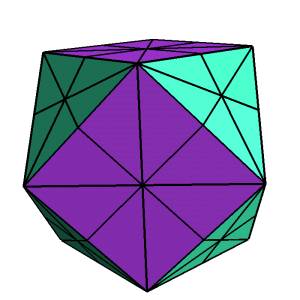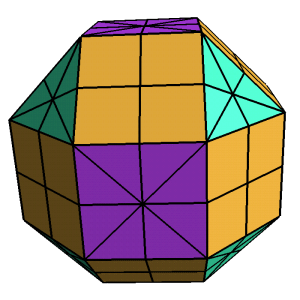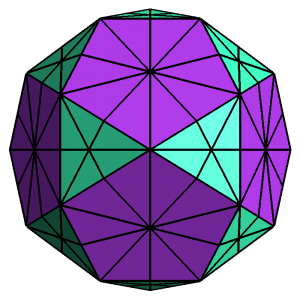Tuesday,  March 2

Space: How do we understand objects in space? Continued..
• Symmetries (Isometries) in the plane compared to those in space- an introduction:
• Spatial Symmetry
The Platonic and Archimedean Solids
.CubeoctahedronRhombicubeoctahedronIcosidodecahedron
• Translations
• Rotations: Center point - central axis
• Reflection :  across line - across plane
• Symmetries of the octhaedron:
• Rotations
• reflections
• rotation- reflection
• Activity. Symmetries of the Cube.
• Isometries in space: products of reflections in space:
• Rotations and translations
• Applications to dance

• Polyhedra and Duality
• In space: Dual polyhedron.
Connected by counting and constructions.
• Vertex - Face
• edge-edge

• Cube- octahedron;
Dodecahedron - icosahedron; tetrahedron.
• Platonic (regular convex polyhedra) and Archimedean (semiregular convex) Solids- on the web!

•  The 5 Platonic Solids
• The Archimedean  Solids in more detail  [Handout!]
•  The 13 Archimedean Solids

• More on The regular and semiregular polyhedra: Check this site!  from Visual geometry pages.
• The Pseudo-Rhombicubeoctahedron.
• Getting familiar with the cube and octahedron with shadows.